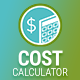# How do I get 1.000 format for the total value?

In order to change format of total value, you'll need to modify plugin’s code.

Please locate line 544 in cc.main.js in plugin’s folder. It should have the following code:

```total = total.toFixed( decimals );
```

Replace the noted line with the following statement:

```total = Math.round(total);
total = total.toString().replace(/\B(?=(\d{3})+(?!\d))/g, ".");```

Make sure to clear cache after making the changes.